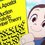# $\int \ln ( \sqrt{x+1} + \sqrt{x} ) dx$ without trigonometry and nice values

Integration:

I watched this video https://www.youtube.com/watch?v=DGpgt8j-nzw in which $\int \ln ( \sqrt{x+1} + \sqrt{x} ) dx$ is computed by using trigonometry. Reading the comments, other solutions by using special functions are shown. However, the final antiderivatives do not use any of these functions, so these are intermediate steps in the most literal interpretation of that phrase. I thought of a solution that sidesteps the need of considering any functions other than those present in the integral:

$\int \ln ( \sqrt{x+1} + \sqrt{x} ) dx$. Let $u = \sqrt{x+1} + \sqrt{x}$. Notice that $\frac{1}{u} = \sqrt{x+1} - \sqrt{x}$. Then $du = \frac{1}{2} \left( \frac{1}{\sqrt{x+1}} + \frac{1}{\sqrt{x}} \right)dx = \frac{1}{2} \frac{u}{\sqrt{x+1} \sqrt{x}}dx$. To write everything in terms of $u$ notice that $u^2 - \frac{1}{u^2} = 4 \sqrt{x+1} \sqrt{x} \implies 2 \sqrt{x+1} \sqrt{x} = \frac{u^4 - 1}{2u^2}$. Thus $du = \frac{u}{\frac{u^4 - 1}{2u^2}}dx = \frac{2u^3}{u^4 - 1} dx$. Going back to the original integral:

$\int \ln ( \sqrt{x+1} + \sqrt{x} ) dx = \int \frac{\ln u}{2u^3} (u^4 - 1)dx = \int \frac{u}{2} \ln u - \frac{ \ln u}{2u^3} du$. Integrating the terms in the integral is now a standard exercise in integration by parts which I will skip. The result is $\frac{1}{8}u^2(2 \ln u - 1) + \frac{2 \log u + 1}{8u^2}$. Giving a final result of:

$\int \ln ( \sqrt{x+1} + \sqrt{x} ) dx = \frac{1}{8}(\sqrt{x+1} + \sqrt{x})^2(2 \ln (\sqrt{x+1} + \sqrt{x}) - 1) + \frac{2 \ln (\sqrt{x+1} + \sqrt{x}) + 1}{8(\sqrt{x+1} + \sqrt{x})^2} + C$

Nice values:

From the form of the antiderivative we can expect this integral to give us nice values whenever $\sqrt{x+1} + \sqrt{x}$ is a nice power of $e$. The equation $\sqrt{x+1} + \sqrt{x} = e^k$ has solution $x= \frac{1}{4} e^{-2 k} (e^{2 k} - 1)^2$. You can obtain this by converting the equation into a normal quadratic equation. The nicest value I was able to find was taking $k=0$ to yield $x = 0$ and $k = \frac{1}{2}$ to yield $x = \frac{(e-1)^2}{4e}$. Then: $\int_{0}^{\frac{(e-1)^2}{4e}} \ln ( \sqrt{x+1} + \sqrt{x} ) dx = \frac{1}{4e}$Note by Leonel Castillo
3 years ago

This discussion board is a place to discuss our Daily Challenges and the math and science related to those challenges. Explanations are more than just a solution — they should explain the steps and thinking strategies that you used to obtain the solution. Comments should further the discussion of math and science.

When posting on Brilliant:

• Use the emojis to react to an explanation, whether you're congratulating a job well done , or just really confused .
• Ask specific questions about the challenge or the steps in somebody's explanation. Well-posed questions can add a lot to the discussion, but posting "I don't understand!" doesn't help anyone.
• Try to contribute something new to the discussion, whether it is an extension, generalization or other idea related to the challenge.

MarkdownAppears as
*italics* or _italics_ italics
**bold** or __bold__ bold
- bulleted- list
• bulleted
• list
1. numbered2. list
1. numbered
2. list
Note: you must add a full line of space before and after lists for them to show up correctly
paragraph 1paragraph 2

paragraph 1

paragraph 2

[example link](https://brilliant.org)example link
> This is a quote
This is a quote
    # I indented these lines
# 4 spaces, and now they show
# up as a code block.

print "hello world"
# I indented these lines
# 4 spaces, and now they show
# up as a code block.

print "hello world"
MathAppears as
Remember to wrap math in $$ ... $$ or $ ... $ to ensure proper formatting.
2 \times 3 $2 \times 3$
2^{34} $2^{34}$
a_{i-1} $a_{i-1}$
\frac{2}{3} $\frac{2}{3}$
\sqrt{2} $\sqrt{2}$
\sum_{i=1}^3 $\sum_{i=1}^3$
\sin \theta $\sin \theta$
\boxed{123} $\boxed{123}$

Sort by:

Here's another way to solve it:

\begin{aligned} \int \ln\left(\sqrt{x+1} + \sqrt x\right) \, dx &=& \frac12 \int \ln\left(\sqrt {x+1} + \sqrt x\right)^2 \, dx \\ &=& \frac12 \int \ln\left(2x + 1 + 2\sqrt{x^2 + x}\right) \, dx \\ &=& \frac12 \int \ln\left[ 2\left(x+ \frac12\right) + 2\sqrt{\left(x + \frac12\right)^2 - \frac14} \; \right] \, dx \\ \end{aligned}

Let $x + \frac12 = \frac12 \sec \theta$, then $\left(x+\frac12\right)^2 - \frac14 = \left(\frac12 \tan\theta\right)^2$ and $\frac{dx}{d\theta} = \frac12\sec\theta\tan\theta$. The integral becomes

\begin{aligned} \int \ln\left(\sqrt{x+1} + \sqrt x\right) \, dx &=& \frac12 \int \ln (\sec \theta + \tan\theta) \cdot \frac12 \sec \theta\tan\theta \, d\theta \\ &=& \frac14 \int \underbrace{\ln (\sec \theta + \tan\theta)}_{=u} \cdot \underbrace{\sec \theta\tan\theta \, d\theta}_{=dv} , \qquad\qquad \text{ Integrate by parts} \\ &=& \frac14 \left (uv - \int v \, du\right) \\ &=& \frac14 \left [ \ln (\sec \theta + \tan\theta) \sec \theta - \int \sec \theta \cdot \dfrac{\sec\theta \tan\theta + \sec^2\theta}{\sec \theta + \tan \theta} \, d\theta \right ] \\ &=& \frac14 \left [ \sec \theta \ln (\sec \theta + \tan\theta) - \int \sec^2\theta \, d\theta \right ] \\ &=& \frac14 \left [ \sec \theta \ln (\sec \theta + \tan\theta) - \tan \theta \right ] +C \\ &=& \frac14 \left [(2x + 1) \ln \left ((2x+1) + \sqrt{(2x+1)^2-1}\; \right) - \sqrt{(2x+1)^2-1} \; \right ] +C \\ &=& \frac{2x+1}4 \ln \left (2x+1 + 2 \sqrt{x^2+x}\; \right) - \frac12 \sqrt{x^2+x} +C \\ &=& \frac{2x+1}2 \ln \left (\sqrt {x+1} + \sqrt x\right) - \frac12 \sqrt{x^2+x} +C \\ \end{aligned}

which is identical to blackpenredpen's final answer.

- 3 years ago

Pi Han Goh, your post does not apply, because the OP expressly asked for the solution to be done without using trigonometry.

- 3 years ago

I did not ask, I was just sharing a solution. If he wants to use this post to post another alternative solution that's okay.

- 3 years ago

OP, your answer is wrong, because you do not include the "+ C."

- 3 years ago

Thank you.

- 3 years ago

Just a thought- How many different sums can be made by adding at least two different numbers from the set of integers {5,6,7,8}?

- 2 years, 9 months ago